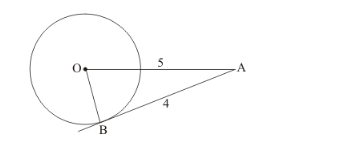# The length of tangent from a point A at a distance of 5 cm from the

Question:

The length of tangent from a point A at a distance of 5 cm from the centre of the circle is 4 cm. What is the radius of the circle?

Solution:

Let us first draw whatever is given for a better understanding of the problem.Let O be the center of the circle and B be the point of contact.

We know that the radius of the circle will be perpendicular to the tangent at the point of contact. Therefore, we haveas a right triangle and we have to apply Pythagoras theorem to find the radius of the triangle.

$\mathrm{OB}^{2}=\mathrm{OA}^{2}-\mathrm{AB}^{2}$

$=5^{2}-4^{2}$

$=25-16$

$=9$

$\mathrm{OB}=\sqrt{9}$

$=3$

Therefore, radius of the circle is 3 cm.